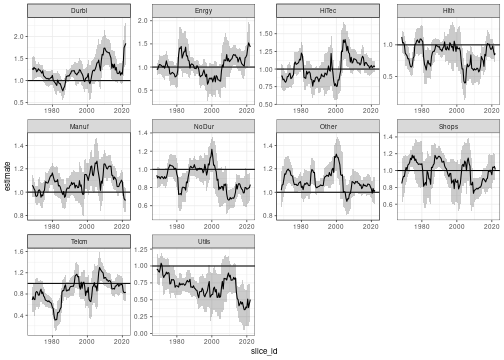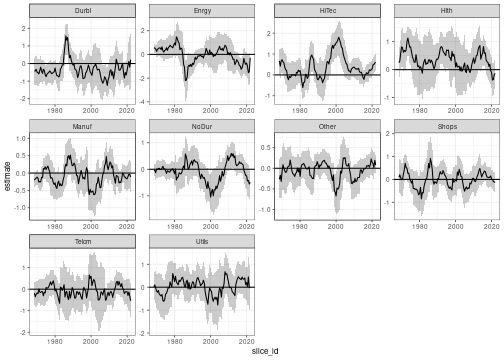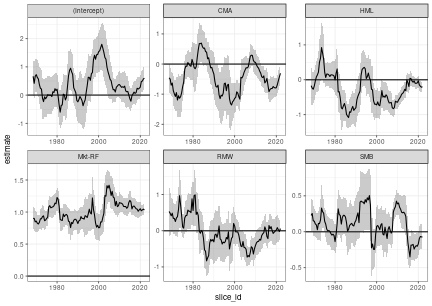# Rolling Window Time Series Regression

In this short tutorial, I show how to calculate a rolling regression on grouped time series data using `tidyfit`. With very few lines of code, we will be able to estimate and analyze a large number of regressions. As usual, begin by loading necessary libraries:

``````library(dplyr); library(tidyr); library(purrr) # Data wrangling
library(ggplot2); library(stringr) # Plotting
library(lubridate)   # Date calculations
library(tidyfit)     # Model fitting
``````

The data set consists monthly industry returns for 10 industries, as well as monthly factor returns for 5 Fama-French factors (data set is available here). The factors are:

1. The market risk premium `Mkt-RF`
2. Size `SMB` (small minus big)
3. Value `HML` (high minus low)
4. Profitability `RMW` (robust minus weak)
5. Investment `CMA` (conservative minus aggressive)

Returns are provided for 10 industries, and excess returns are calculated by subtracting the risk free rate from the monthly industry returns:

``````data <- Factor_Industry_Returns
data <- data %>%
mutate(Date = ym(Date)) %>%         # Parse dates
mutate(Return = Return - RF) %>%    # Excess return
select(-RF)
data
#> # A tibble: 7,080 × 8
#>    Date       Industry Return `Mkt-RF`   SMB   HML   RMW   CMA
#>    <date>     <chr>     <dbl>    <dbl> <dbl> <dbl> <dbl> <dbl>
#>  1 1963-07-01 NoDur     -0.76    -0.39 -0.44 -0.89  0.68 -1.23
#>  2 1963-08-01 NoDur      4.64     5.07 -0.75  1.68  0.36 -0.34
#>  3 1963-09-01 NoDur     -1.96    -1.57 -0.55  0.08 -0.71  0.29
#>  4 1963-10-01 NoDur      2.36     2.53 -1.37 -0.14  2.8  -2.02
#>  5 1963-11-01 NoDur     -1.4     -0.85 -0.89  1.81 -0.51  2.31
#>  6 1963-12-01 NoDur      2.52     1.83 -2.07 -0.08  0.03 -0.04
#>  7 1964-01-01 NoDur      0.49     2.24  0.11  1.47  0.17  1.51
#>  8 1964-02-01 NoDur      1.61     1.54  0.3   2.74 -0.05  0.9
#>  9 1964-03-01 NoDur      2.77     1.41  1.36  3.36 -2.21  3.19
#> 10 1964-04-01 NoDur     -0.77     0.1  -1.59 -0.58 -1.27 -1.04
#> # ℹ 7,070 more rows
``````

The aim of the analysis is to calculate rolling window factor betas using a regression for each industry. To fit a regression for each industry, we simply group the data:

``````data <- data %>%
group_by(Industry)
``````

Let’s verify that this fits individual regressions:

``````mod_frame <- data %>%
regress(Return ~ CMA + HML + `Mkt-RF` + RMW + SMB, m("lm"))

mod_frame
#> # A tibble: 10 × 7
#>    Industry model estimator_fct `size (MB)` grid_id  model_object settings
#>    <chr>    <chr> <chr>               <dbl> <chr>    <list>       <list>
#>  1 Durbl    lm    stats::lm           0.453 #0010000 <tidyFit>    <tibble>
#>  2 Enrgy    lm    stats::lm           0.453 #0010000 <tidyFit>    <tibble>
#>  3 HiTec    lm    stats::lm           0.453 #0010000 <tidyFit>    <tibble>
#>  4 Hlth     lm    stats::lm           0.453 #0010000 <tidyFit>    <tibble>
#>  5 Manuf    lm    stats::lm           0.453 #0010000 <tidyFit>    <tibble>
#>  6 NoDur    lm    stats::lm           0.453 #0010000 <tidyFit>    <tibble>
#>  7 Other    lm    stats::lm           0.453 #0010000 <tidyFit>    <tibble>
#>  8 Shops    lm    stats::lm           0.453 #0010000 <tidyFit>    <tibble>
#>  9 Telcm    lm    stats::lm           0.453 #0010000 <tidyFit>    <tibble>
#> 10 Utils    lm    stats::lm           0.453 #0010000 <tidyFit>    <tibble>
``````

The model frame now contains 10 regressions estimated using `stats::lm`. We may want to fit the regression using a heteroscedasticity and autocorrelation consistent (HAC) estimate of the covariance matrix, since we are working with financial time series. This can be done by passing the additional argument to `m(...)`:^[Note that the `...` in `m()` passes arguments to the underlying method (i.e. `stats::lm`). However in some cases `tidyfit` adds convenience functions, such as the ability to pass a `vcov.` argument as used in `coeftest` for `lm`.]

``````mod_frame_hac <- data %>%
regress(Return ~ CMA + HML + `Mkt-RF` + RMW + SMB, m("lm", vcov. = "HAC"))

mod_frame_hac
#> # A tibble: 10 × 7
#>    Industry model estimator_fct `size (MB)` grid_id  model_object settings
#>    <chr>    <chr> <chr>               <dbl> <chr>    <list>       <list>
#>  1 Durbl    lm    stats::lm           0.453 #0010000 <tidyFit>    <tibble>
#>  2 Enrgy    lm    stats::lm           0.453 #0010000 <tidyFit>    <tibble>
#>  3 HiTec    lm    stats::lm           0.453 #0010000 <tidyFit>    <tibble>
#>  4 Hlth     lm    stats::lm           0.453 #0010000 <tidyFit>    <tibble>
#>  5 Manuf    lm    stats::lm           0.453 #0010000 <tidyFit>    <tibble>
#>  6 NoDur    lm    stats::lm           0.453 #0010000 <tidyFit>    <tibble>
#>  7 Other    lm    stats::lm           0.453 #0010000 <tidyFit>    <tibble>
#>  8 Shops    lm    stats::lm           0.453 #0010000 <tidyFit>    <tibble>
#>  9 Telcm    lm    stats::lm           0.453 #0010000 <tidyFit>    <tibble>
#> 10 Utils    lm    stats::lm           0.453 #0010000 <tidyFit>    <tibble>
``````

A rolling window regression can be estimated by specifying an appropriate cross validation method. `tidyfit` uses the `rsample` package from the `tidymodels` suite to create cross validation slices. Apart from the classic CV methods (vfold, loo, expanding window, train/test split), this also includes sliding functions (`sliding_window`, `sliding_index` and `sliding_period`), as well as `bootstraps`. The sliding functions do not generate a validation slice, but only create rolling window training slices. We will be using `sliding_index`, which works well with date indices. See `?rsample::sliding_index` for specifics.^[It is important to note that `sliding_index` can not be used to select hyperparameters since no test slices are produced. To perform time series CV, use the `rolling_origin` setting, which is similar, but produces train and test splits.]

Since the method `lm` has no hyperparameters, it is not passed to the cross validation loop by default, since this would create unnecessary computational overhead. To ensure that models are fitted on train slices, we need to add the additional argument `.force_cv = TRUE`. Furthermore, the CV slices are typically not returned but only used to select optimal hyperparameters. To return slices set `.return_slices = TRUE`:

``````mod_frame_rolling <- data %>%
regress(Return ~ CMA + HML + `Mkt-RF` + RMW + SMB,
m("lm", vcov. = "HAC"),
.cv = "sliding_index", .cv_args = list(lookback = years(5), step = 6, index = "Date"),
.force_cv = TRUE, .return_slices = TRUE)
``````

The `.cv_args` are arguments passed directly to `rsample::sliding_index`. We are using a 5 year window (`lookback = years(5)`), and skipping 6 months between each window to reduce the number of models fitted (`step = 6`).

The model frame now contains models for each slice for each industry. The `slice_id` provides the valid date for the respective slices. Betas can be extracted using the built-in `coef` function:

``````df_beta <- coef(mod_frame_rolling)
``````
``````df_beta
#> # A tibble: 6,480 × 6
#> # Groups:   Industry, model 
#>    Industry model term        estimate slice_id   model_info
#>    <chr>    <chr> <chr>          <dbl> <chr>      <list>
#>  1 Durbl    lm    (Intercept)  -0.434  1968-07-01 <tibble [1 × 3]>
#>  2 Durbl    lm    CMA           0.0258 1968-07-01 <tibble [1 × 3]>
#>  3 Durbl    lm    HML           0.661  1968-07-01 <tibble [1 × 3]>
#>  4 Durbl    lm    Mkt-RF        1.28   1968-07-01 <tibble [1 × 3]>
#>  5 Durbl    lm    RMW           0.898  1968-07-01 <tibble [1 × 3]>
#>  6 Durbl    lm    SMB          -0.150  1968-07-01 <tibble [1 × 3]>
#>  7 Durbl    lm    (Intercept)  -0.423  1969-01-01 <tibble [1 × 3]>
#>  8 Durbl    lm    CMA           0.235  1969-01-01 <tibble [1 × 3]>
#>  9 Durbl    lm    HML           0.569  1969-01-01 <tibble [1 × 3]>
#> 10 Durbl    lm    Mkt-RF        1.25   1969-01-01 <tibble [1 × 3]>
#> # ℹ 6,470 more rows
``````

In order to plot the betas, we will add confidence bands. HAC standard errors are nested in `model_info` in the coefficients frame:

``````df_beta <- df_beta %>%
unnest(model_info) %>%
mutate(upper = estimate + 2 * std.error, lower = estimate - 2 * std.error)
``````
``````df_beta
#> # A tibble: 6,480 × 10
#> # Groups:   Industry, model 
#>    Industry model term      estimate slice_id std.error statistic  p.value upper
#>    <chr>    <chr> <chr>        <dbl> <chr>        <dbl>     <dbl>    <dbl> <dbl>
#>  1 Durbl    lm    (Interce…  -0.434  1968-07…     0.383   -1.13   2.62e- 1 0.332
#>  2 Durbl    lm    CMA         0.0258 1968-07…     0.262    0.0985 9.22e- 1 0.550
#>  3 Durbl    lm    HML         0.661  1968-07…     0.239    2.77   7.68e- 3 1.14
#>  4 Durbl    lm    Mkt-RF      1.28   1968-07…     0.124   10.3    2.00e-14 1.52
#>  5 Durbl    lm    RMW         0.898  1968-07…     0.378    2.37   2.11e- 2 1.65
#>  6 Durbl    lm    SMB        -0.150  1968-07…     0.133   -1.13   2.65e- 1 0.116
#>  7 Durbl    lm    (Interce…  -0.423  1969-01…     0.396   -1.07   2.90e- 1 0.369
#>  8 Durbl    lm    CMA         0.235  1969-01…     0.264    0.889  3.78e- 1 0.764
#>  9 Durbl    lm    HML         0.569  1969-01…     0.243    2.34   2.29e- 2 1.06
#> 10 Durbl    lm    Mkt-RF      1.25   1969-01…     0.150    8.33   2.52e-11 1.55
#> # ℹ 6,470 more rows
#> # ℹ 1 more variable: lower <dbl>
``````

Now, with all the pieces in place, we can plot the results. Let’s begin by examining the market beta for each industry using `ggplot2`:

``````df_beta %>%
mutate(slice_id = as.Date(slice_id)) %>%
filter(term == "Mkt-RF") %>%
ggplot(aes(slice_id)) +
geom_hline(yintercept = 1) +
facet_wrap("Industry", scales = "free") +
geom_ribbon(aes(ymax = upper, ymin = lower), alpha = 0.25) +
geom_line(aes(y = estimate)) +
theme_bw(8)
``````Or plotting risk-adjusted return (alpha) given by the intercept:

``````df_beta %>%
mutate(slice_id = as.Date(slice_id)) %>%
filter(term == "(Intercept)") %>%
ggplot(aes(slice_id)) +
geom_hline(yintercept = 0) +
facet_wrap("Industry", scales = "free") +
geom_ribbon(aes(ymax = upper, ymin = lower), alpha = 0.25) +
geom_line(aes(y = estimate)) +
theme_bw(8)
``````Or plotting all parameters for the HiTec industry:

``````df_beta %>%
mutate(slice_id = as.Date(slice_id)) %>%
filter(Industry == "HiTec") %>%
ggplot(aes(slice_id)) +
geom_hline(yintercept = 0) +
facet_wrap("term", scales = "free") +
geom_ribbon(aes(ymax = upper, ymin = lower), alpha = 0.25) +
geom_line(aes(y = estimate)) +
theme_bw(8)
``````While there are certainly very interesting insights to be had here, the aim of this walk-through is not to interpret these results, but rather to demonstrate how a complex piece of analysis can be done very efficiently using the `tidyfit` package.

In a follow-up article found here, I compare the results in the plot above to a time-varying parameter regression to show how more robust methods can be used to avoid window effects in the rolling sample and to shrink some coefficients to constant values.